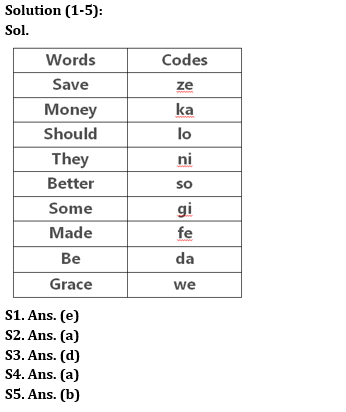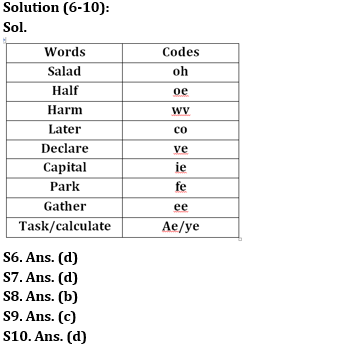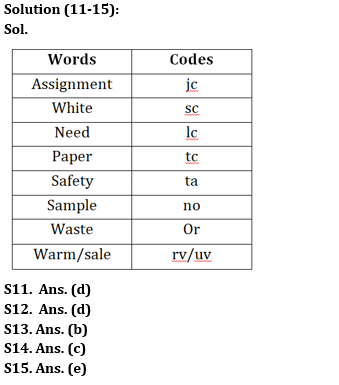Latest Banking jobs   »

# Reasoning Ability Quiz For IBPS RRB PO Prelims 2022- 31st May

Directions (1-5): Study the information given below and answer the questions based on it.
In a certain code language,
‘Should save some money’ is coded as ‘ze lo ka gi’
‘They made better money’ is coded as ‘fe ka so ni’
‘They should be better’ is coded as ‘ni lo da so’
‘Be better save grace’ is coded as ‘we so ze da’

Q1. What does the code ‘ze’ stand for?
(a) Some
(b) Should
(c) Be
(d) Grace
(e) Save

Q2. Which of the following is the code of ‘better’?
(a) So
(b) We
(c) Ze
(d) Lo
(e) Fe

Q3. ‘Grace of money’ may be coded as?
(a) ka da fe
(b) we ka so
(c) ja da wo
(d) ka we yo
(e) ja ka je

Q4. What does the code ‘gi fe’ stand for?
(b) Should better
(c) Be money
(e) Save some

Q5. Which of the following is the code of ‘grace’?
(a) da
(b) we
(c) ze
(d) lo
(e) None of these

Directions (6-10): Study the following data carefully and answer the questions accordingly.
In a certain code language-
“Salad half harm later” is written as “co oe oh wv”.
“Declare capital park salad” is written as “fe oh ve ie”.
“Harm declare gather later” is written as “ve co wv ee”.
“Later task park calculate” is written as “fe ae ye co”.

Q6. What will be the possible code for “gather white capital”?
(a) ie ee fe
(b) ie oh co
(c) ee ve cf
(d) ie ee bh
(e) fe ie ve

Q7. If “salad task declare” is coded as “ae oh ve”, then what will be the code for “calculate”?
(a) co
(b) fe
(c) ae
(d) ye
(e) None of these

Q8. What is the code for “park”?
(a) ae
(b) fe
(c) co
(d) wv
(e) None of these

Q9. Which of the following is coded as ‘oe’?
(a) Harm
(b) Later
(c) Half
(d) Declare
(e) None of these

Q10. If “later gain harm” is written as “wv co te”, then what will be the code for “gain”?
(a) oh
(b) wv
(c) co
(d) te
(e) None of these

Directions (11-15): Study the following information carefully and answer the questions accordingly.
In a certain code language:
‘Assignment white need paper’ is coded as ‘lc jc tc sc’,
‘Safety white paper’ is coded as ‘sc ta tc’,
‘Assignment white sample waste’ is coded as ‘jc sc or no’,
‘Warm sale waste’ is coded as ‘or uv rv’.

Q11. What is the code for ‘need’?
(a) tc
(b) sc
(c) jc
(d) lc
(e) None of these

Q12. What is the code for ‘white’?
(a) lc
(b) jc
(c) ta
(d) sc
(e) None of these

Q13. What is the code for ‘paper’?
(a) lc
(b) tc
(c) ta
(d) sc
(e) None of these

Q14. What could be the code for ‘waste sample safety’?
(a) ta or tc
(b) jc no or
(c) ta no or
(d) no vu ta
(e) None of these

Q15. Which of the following is coded as ‘uv’?
(a) Waste
(b) Sale
(c) Paper
(d) Warm
(e) Can’t be determined

Solutions#### Congratulations!